International Tables for Crystallography (2006). Vol. D, ch. 2.2, pp. 294-313https://doi.org/10.1107/97809553602060000639

## Contents

• 2.2. Electrons  (pp. 294-313)
• 2.2.1. Introduction  (p. 294) | html | pdf |
• 2.2.2. The lattice  (p. 294) | html | pdf |
• 2.2.2.1. The direct lattice and the Wigner–Seitz cell  (p. 294) | html | pdf |
• 2.2.2.2. The reciprocal lattice and the Brillouin zone  (p. 294) | html | pdf |
• 2.2.3. Symmetry operators  (pp. 294-295) | html | pdf |
• 2.2.3.1. Transformation of functions  (pp. 294-295) | html | pdf |
• 2.2.3.2. Transformation of operators  (p. 295) | html | pdf |
• 2.2.3.3. The Seitz operators  (p. 295) | html | pdf |
• 2.2.3.4. The important groups and their first classification  (p. 295) | html | pdf |
• 2.2.4. The Bloch theorem  (pp. 295-296) | html | pdf |
• 2.2.4.1. A simple quantum-mechanical derivation  (pp. 295-296) | html | pdf |
• 2.2.4.2. Periodic boundary conditions  (p. 296) | html | pdf |
• 2.2.4.3. A simple group-theoretical approach  (p. 296) | html | pdf |
• 2.2.5. The free-electron (Sommerfeld) model  (p. 297) | html | pdf |
• 2.2.6. Space-group symmetry  (pp. 297-298) | html | pdf |
• 2.2.6.1. Representations and bases of the space group  (pp. 297-298) | html | pdf |
• 2.2.6.2. Energy bands  (p. 298) | html | pdf |
• 2.2.7. Thevector and the Brillouin zone  (p. 298) | html | pdf |
• 2.2.7.1. Various aspects of thevector  (p. 298) | html | pdf |
• 2.2.7.2. The Brillouin zone (BZ)  (p. 298) | html | pdf |
• 2.2.7.3. The symmetry of the Brillouin zone  (p. 298) | html | pdf |
• 2.2.8. Bloch functions  (p. 299) | html | pdf |
• 2.2.9. Quantum-mechanical treatment  (pp. 299-300) | html | pdf |
• 2.2.9.1. Exchange and correlation treatment  (p. 299) | html | pdf |
• 2.2.9.2. The choice of basis sets and wavefunctions  (p. 299) | html | pdf |
• 2.2.9.3. The form of the potential  (p. 299) | html | pdf |
• 2.2.9.4. Relativistic effects  (p. 300) | html | pdf |
• 2.2.10. Density functional theory  (pp. 300-301) | html | pdf |
• 2.2.11. Band-theory methods  (pp. 301-303) | html | pdf |
• 2.2.11.1. LCAO (linear combination of atomic orbitals)  (p. 301) | html | pdf |
• 2.2.11.2. TB (tight binding)  (pp. 301-302) | html | pdf |
• 2.2.11.3. The pseudo-potential schemes  (p. 302) | html | pdf |
• 2.2.11.4. APW (augmented plane wave) and LAPW methods  (p. 302) | html | pdf |
• 2.2.11.5. KKR (Korringa–Kohn–Rostocker) method  (p. 302) | html | pdf |
• 2.2.11.6. LMTO (linear combination of muffin-tin orbitals) method  (p. 302) | html | pdf |
• 2.2.11.7. CP (Car–Parrinello) method  (p. 302) | html | pdf |
• 2.2.11.8. Order N schemes  (pp. 302-303) | html | pdf |
• 2.2.12. The linearized augmented plane wave method  (pp. 303-304) | html | pdf |
• 2.2.13. The local coordinate system  (pp. 304-305) | html | pdf |
• 2.2.13.1. Crystal harmonics  (pp. 304-305) | html | pdf |
• 2.2.13.2. Interpretation for bonding  (p. 305) | html | pdf |
• 2.2.14. Characterization of Bloch states  (pp. 305-307) | html | pdf |
• 2.2.14.1. Characterization by group theory  (p. 305) | html | pdf |
• 2.2.14.2. Energy regions  (p. 305) | html | pdf |
• 2.2.14.3. Decomposition according to wavefunctions  (pp. 305-306) | html | pdf |
• 2.2.14.4. Localized versus itinerant electrons  (p. 306) | html | pdf |
• 2.2.14.5. Spin polarization  (p. 306) | html | pdf |
• 2.2.14.6. The density of states (DOS)  (pp. 306-307) | html | pdf |
• 2.2.15. Electric field gradient tensor  (pp. 307-310) | html | pdf |
• 2.2.15.1. Introduction  (p. 307) | html | pdf |
• 2.2.15.2. EFG conversion formulas  (pp. 307-308) | html | pdf |
• 2.2.15.3. Theoretical approach  (pp. 308-310) | html | pdf |
• 2.2.16. Examples  (pp. 310-312) | html | pdf |
• 2.2.16.1. F.c.c. copper  (pp. 310-311) | html | pdf |
• 2.2.16.2. The rutile TiO 2   (p. 311) | html | pdf |
• 2.2.16.3. Core electron spectra  (p. 312) | html | pdf |
• 2.2.17. Conclusion  (p. 312) | html | pdf |
• References | html | pdf |
• Figures
• Fig. 2.2.5.1. Plane waves  (p. 297) | html | pdf |
• Fig. 2.2.7.1. The Brillouin zone (BZ) and the irreducible wedge of the BZ for the f.c.c. direct lattice  (p. 298) | html | pdf |
• Fig. 2.2.12.1. Schematic partitioning of the unit cell into atomic spheres (I) and an interstitial region (II)  (p. 303) | html | pdf |
• Fig. 2.2.14.1. Relativistic radial wavefunctions (large component) of the uranium atom  (p. 306) | html | pdf |
• Fig. 2.2.15.1. Unit cell of the high-temperature superconductor YBa 2 Cu 3 O 7 with four non-equivalent oxygen sites  (p. 309) | html | pdf |
• Fig. 2.2.16.1. Character of energy bands of f.c.c. copper in thedirection  (p. 310) | html | pdf |
• Fig. 2.2.16.2. Decomposition of the Cu d bands into theand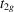manifold  (p. 311) | html | pdf |
• Fig. 2.2.16.3. The local coordinate system in rutile for titanium (small spheres) and oxygen (large spheres)  (p. 311) | html | pdf |
• Fig. 2.2.16.4. Schematic transitions in X-ray emission and absorption spectra  (p. 311) | html | pdf |
• Tables
• Table 2.2.13.1. Picking rules for the local coordinate axes and the corresponding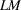combinations (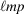) of non-cubic groups taken from Kurki-Suonio (1977)  (p. 304) | html | pdf |
• Table 2.2.13.2. LM combinations of cubic groups as linear cominations of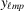's (given in parentheses)  (p. 304) | html | pdf |
• Table 2.2.15.1. Partial O 2 p charges (in electrons) and electric field gradient tensor O EFG (in 10 21  V m −2 ) for YBa 2 Cu 3 O 7   (p. 309) | html | pdf |
• Table 2.2.16.1.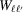factors for X-ray emission spectra showing the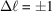selection rule  (p. 312) | html | pdf |